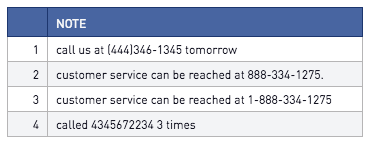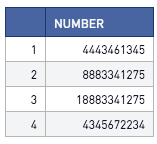Extract a phone number from a string in Redshift

Sometimes, phone numbers don't come in a format that easy for extraction. It requires a little bit of magic with Regex expressions in Redshift to get what you're looking for

Let's say I have this table, called "comments," as my underlying dataset. I want to extract the phone numbers from here.This is the script I used:

select
regexp_substr(replace(replace(replace(note, '(', ''), ')', ''), '-', ''), '\\d{10}\\d?') as number
from

And this is the result:How this works:

1. We first remove the parenthesis and the hyphens from the expression by using the REPLACE function (we are replacing these characters with blank strings) replace(replace(replace(note, '(', ''), ')', ''), '-', '')
2. Then, we use REGEXP_SUBSTR, and use POSIX operators to define the pattern we are looking for... Here, we want a string that is 10 or 11 digits (in case there's a digit for the country code). The pattern-matching expression for this would be '\\d{10}\\d?'

Breaking down the pattern matching expression:

\\d - this is code for "digit"

{10} this tells us how many instances of the previous character must appear in a row. So, '\\d{10}' is 10 digits, and '\\d{3} is 3 digits, and so on

\\d? This tells us there might be an additional digit. The question mark matches 0 or 1 occurrences of the character specified immediately before it

Any improvements or alternative methods you'd suggest for extracting a phone number?